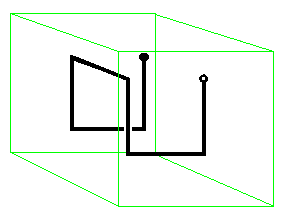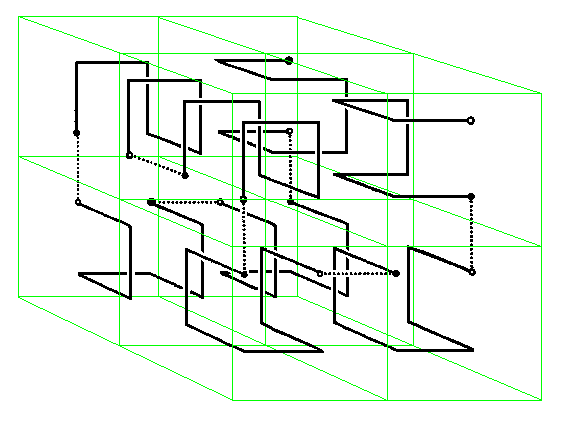# A Cube-filling Hilbert Curve

## William Gilbert

Pure Mathematics Department, University of Waterloo, Ontario, Canada

### Mathematical Intelligencer 6(3) (1984), page 78In 1891 David Hilbert gave a simple construction of a Peano curve whose limit filled a square. We exhibit a direct generalization of Hilbert's curve that fills a cube. The first three iterates of this curve are shown. In constructing one iterate from the previous one, note that the direction of the curve determines the orientation of the smaller cubes inside the larger one.

The initial stage of this three dimensional curve can be considered as coming from the 3-bit reflected Gray code which traverses the 3-digit binary strings in such a way that each string differs from its predecessor in a single position by the addition or subtraction of 1. The kth iterate could be considered a a generalized Gray code on the Cartesian product set {0,1,2,...,2^k-1}^3.

The n-bit reflected binary Gray code will describe a path on the edges of an n-dimensional cube that can be used as the initial stage of a Hilbert curve that will fill an n-dimensional cube.

### Reference

E. N. Gilbert, (1958) Gray codes and the paths on the n-cube. Bell System Tech. J. 37 pp. 815-826.

## Notes on A Cube-filling Hilbert Curve

This cube-filling Hilbert curve was also produced independently by
• R. J. Stevens, A. F. Lehar, and F. H. Perston, Manipulation and presentation of multidimensional image data using the Peano scan. IEEE Trans. on Pattern Analysis and Machine Intelligence, PAMI-5(5) (1983) pp. 520-526.